﻿ Schrödinger equation has No exact solution in Helium

# Schrödinger equation  is false.

## Schrödinger's atom = parallel worlds !?

### [ An electron is everywhere at the same time in quantum world ? ]

(Fig.1)  Schrödinger's hydrogen = an electron everywhere !?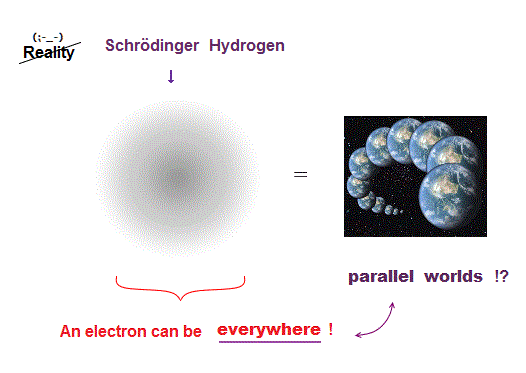Quantum mechanics uses Schrödinger equation, where a single electron can be everywhere at the same time in parallel worlds.

Quantum mechanics tries to apply these unreal parallel worlds to all atoms !

But this "parallel-world" nature of Schrödinger equation does Not work in multi-electron atoms.

Here we prove quantum mechanics is wrong.

## No electron  in quantum mechanics ?

### [ There is No clear electron, No motion in Schrödinger atom ! ]

(Fig.2)  Just vague electron cloud in quantum atom ↓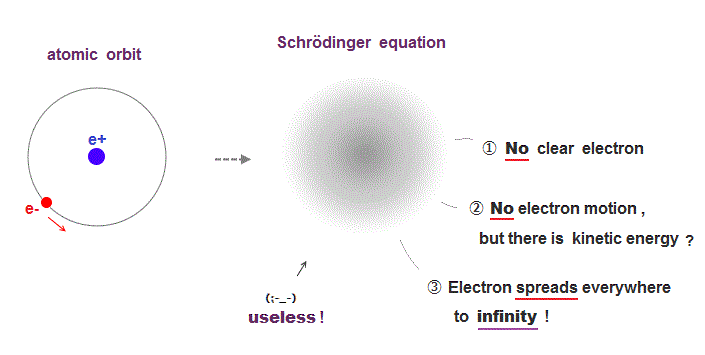Quantum mehanics can not descibe real electrons.
It gives only vague electron cloud spreading over all space to infinity.

Strangely, though Schrödinger equation has kinetic energy, its electron is Not moving "realistically" !

This unphysical nature of quantum mechanics = "No clear electron, No electron's motion" causes serious problem in multi- electron atoms.

Because it's unreal.

## Electron "everywhere"  in quantum mechanics !

### [ Schrödinger wavefunction spreads all space ← electron everywhere !? ]

(Fig.3)  ↓ Only one-electron hydrogen has solution in quantum mechanics.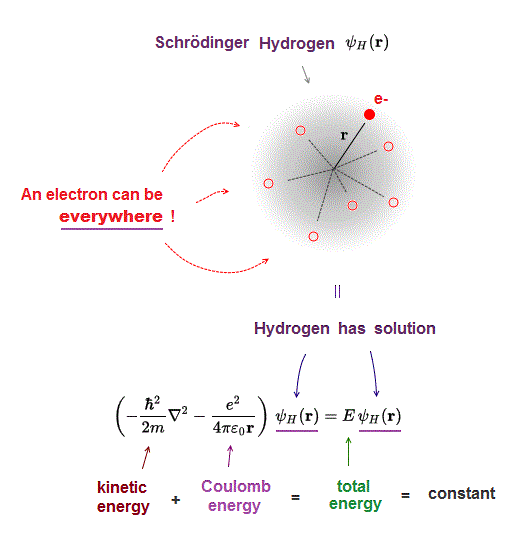Only one-electron hydrogen atom has solution in Schrödinger equation.

Hydrogen wavefunction spreads all space, so in all space, the electron's total energy must be conserved at constant E.

Coulomb energy term must be cancelled out by kinetic energy to give constant total energy in all space.

This is impossible in multi-electron Schrödinger atoms.

## Quantum mechanical Helium can Not find exact solution.

### [ Schrödinger probability wavefunction of each electron must always spread all over the space = a single electron is everywhere !? ]

(Fig.4)   Two hydrogen wavefunctions → Helium ?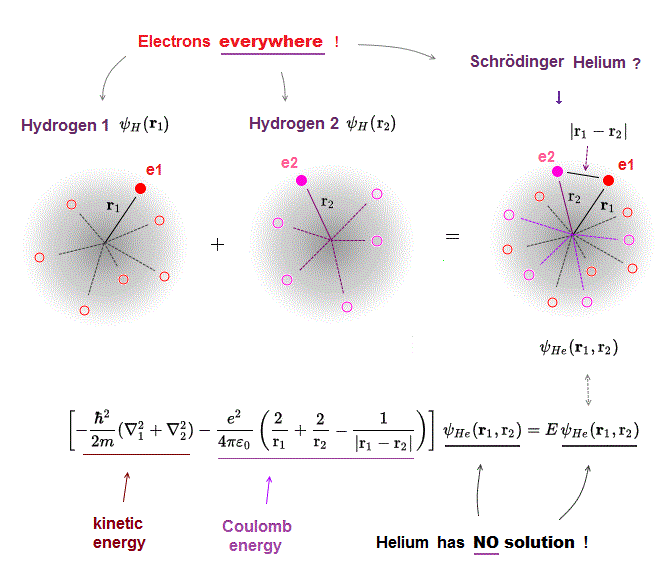All Schrödinger equations for multi-electron atoms such as two-electron helium are known to be unsolvable.

Basically, quantum mechanics tries to waste time in choosing fake approximate solution or trial wavefunction as a fictitious helium wavefunction (= the simplest one is a fititious helium wavefunction consists of two hydrogen 1s atomic wavefunctions ) and integrating it to obtain fake average total energy E instead of solving the unsolvable Schrödinger equation for helium.

We can prove Schrödinger equations for multi-electron atoms such as helium can never find true solutions, no matter what fake trial wavefunctions are chosen.

This is due to the always-spreading probability wavefunction of quantum mechanics where a single electron must unrealistically exist everywhere regardless of the other electrons' positions.

## Distance between two electrons of Schrödinger Helium can be unrealistically changeable.

### [ Distance (= |r1-r2| ) between two electrons (= e1 and e2 ) can be changed independently of other distance variables r1 and r2. ]

(Fig.5)  ↓ Three Coulomb terms can be changed independently !?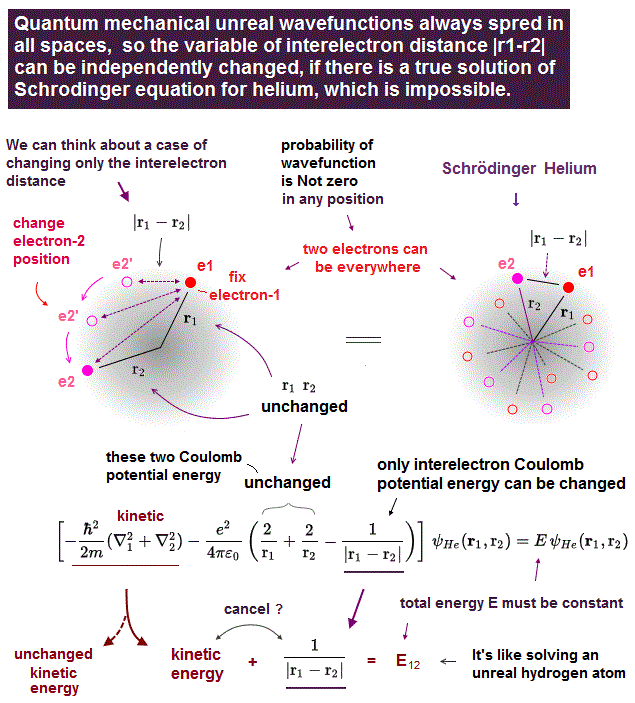Each single electron's probability wavefunction of quantum mechanics must always spread all over the place like a vague electron cloud with No boundaries.

Actually, the hydrogen atomic exact wavefunction obtained by solving its Schrödinger equation contains the exponential function which spreads out throughout the entire space to infinite points ( r → ∞ ).

Helium's approximate wavefunctions must also contain the exponential wavefunctions ( this p.2 ) whose electron's probability spreads all over the places (= electron's probabiliy can decrease or increase, but in No places the electron's probability becomes completely zero except for several node points ).

Because if the probability wavefunction suddenly discontinues and disappears at some point, the electron's kinetic energy term, which is equal to the derivative of wavefunction, unrealistically diverges to infinity (= kinetic energy differential gives infinite kinetic energies at the discontinuous wavefunction point where the slope is infinitely steep ).

To avoid such an unrealistic infinite kinetic energies, Schrödinger's probability wavefunction must always spread all over the place smoothly and continuously, as shown in the exponential wavefunction.

This unrealistic quantum mechanical probability wavefunction or spreading electron's cloud disproves quantum mechanics itself.

Schrödinger's equation for helium's two electrons (= e1 and e2 ) consists of two kinetic energy term expressed as the differential operators and three Coulomb potential energy terms ( this p.2 ).

These three Coulomb potential energy terms are Coulomb attractive energy between the electron-1 and the helium nucleus (= distance r1 ), Coulomb attractive energy between the electron-2 and the nucleus (= distance r2 ), and Coulomb repulsive energy between two electrons-1 and -2 (= distance |r1-r2| or r12 ).

In the upper figure, we can freely change the position of the electron-1 so that only the distance (= |r1-r2| or r12 ) between two electrons e1 and e2 is changed while other distance (= r1 ) between electron-1 (=e1 ) and the nucleus and the distance (= r2 ) between electron-2 and the nucleus are unchanged.

This means we can freely change only one Coulomb repulsive energy term between two electrons (= only e2/4πε |r1-r2| Coulomb energy can be changed ) while keeping other two Coulomb attractive energy terms unchanged (= = -e2/4πε r1 and -e2/4πε r2 are unchanged and fixed )

## Each Coulomb potential energy term is freely changeable in unscientific Schrödinger's Helium.

### [ Artificially changing only one variable r1 ( or r2 ) and changing only one Coulomb potential energy term is possible in quantum mechanical helium. ]

(Fig.5')  ↓ Only one variable or distance r1 (or r2 ) can be freely changed in quantum mechanical helium atoms.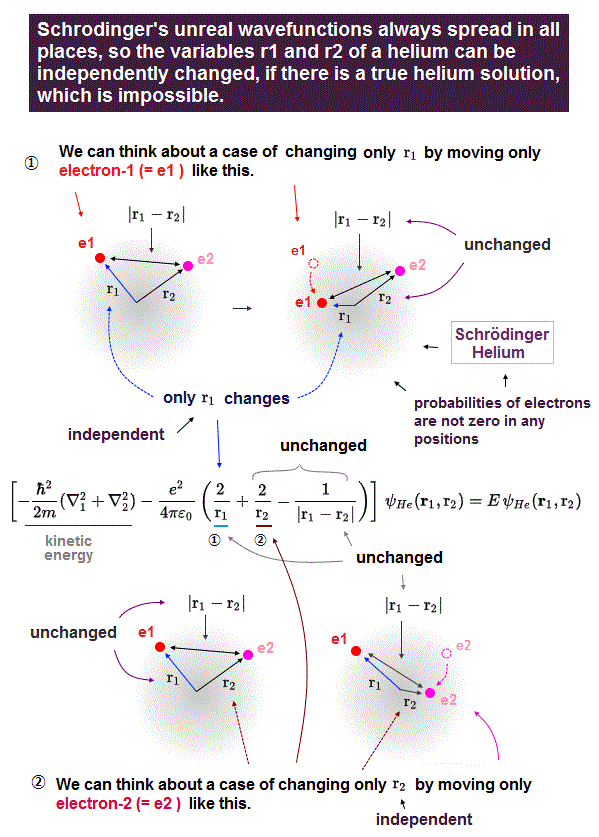As shown in Fig.5', we can freely change only one variable or distance (= r1 ) between the electron-1 and the helium nucleus, while other distance variables (= r2 amd |r1-r2 | ) are kept unchanged.

So in quantum mechanical helium, three distance variables and their three Coulomb energy terms are all free parameters changeable independently of the other two variables or Coulomb potential energy terms, which unscientific quantum mechanical atomic property causes serious problems.

## Quantum mechanical Helium must split into three unreal independent hydrogen atoms to have the exact solution.

### [ Helium must split into three unreal hydrogens, if Helium had exact solution for Schrödinger equation, so quantum mechanics is false. ]

(Fig.6)  ↓ Helium = three unreal hydrogens ? ← Schrödinger is wrong !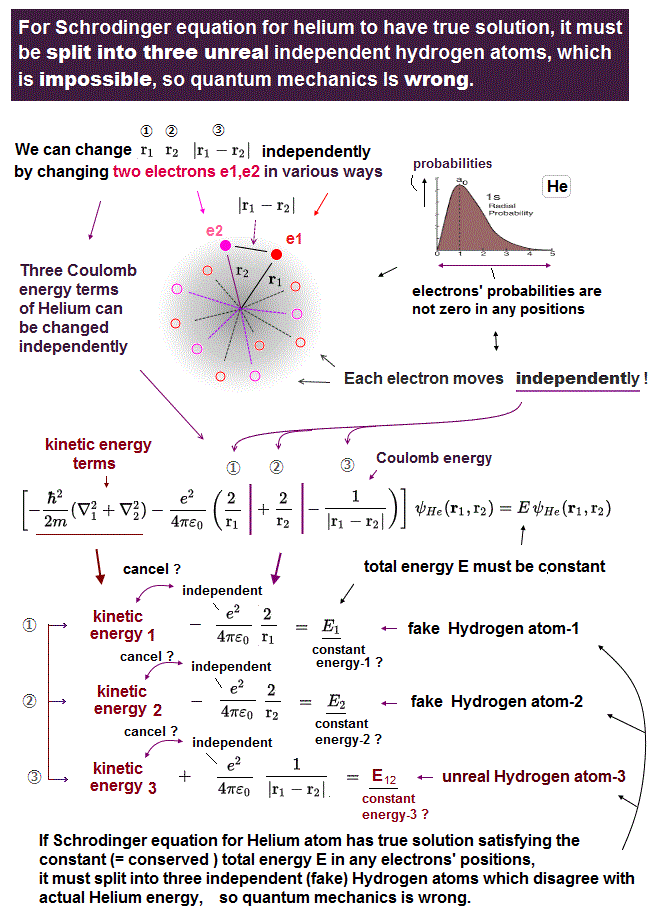Quantum mechanics has to solve Schrödinger equations in order to know the exact atomic wavefunctions or solutions.

In all Schrödinger equations, the sum of electrons' kinetic energies and Coulomb potential energies is equal to the total energy E which value must be constant and conserved ( this p.2 ).

↑ Atomic energy E is described by time-independent Schrödinger equation, so the total energy E must be constant and conserved also in quantum mechanical rule ( this p.2-4th-paragraph,  this 7th-paragraph ).

In electrons' positions where Coulomb potential energies become higher, the electrons' kinetic energies must be lower to conserve the constant total energy E inside a atom and molecule ( this p.2-1st-paragraph,  this p.1-last ).

Actually, the exact wavefunction and energy levels of the one-electron hydrogen atoms are obtained by solving its Schrödinger equation (= one-electron hydrogen atom is the only atom which can solve Schrödinger equation whose energy levels are just equal to Bohr's realistic atom ) under the condition of the constant and conserved total energy E ( this p.3 ).

So if quantum mechanics was right, any other multi-electron atoms and molecules must find their exact wavefunctions and solutions by legitimately solving their Schrödinger equations under the condition of the constant total energy E value regardless of electrons' positions.

But all Schrödinger equations for multi-electron atoms such as helium and molecules are known to be unsolvable, so physicists have to artificially choose trial wavefunctions with many freely-adjustable parameters as fake approximate solutions and integrate them to get fake average energies E (= Not a true single ground-state energy ) instead of solving Schrödinger equations, which quantum mechanical (variational) methods just choosing fake solutions out of infinite choices have No ability to predict any atomic true wavefunctions or energies.  ← completely useless.

Here, we prove No Schrödinger equations for multi-electron atoms and molecules can have their exact solutions conserving the constant total energy E, no matter what fictitious trial wavefunctions physicists artificially choose out of infinite choices.

Hence, quantum mechanics can be proved to be intrinsically false.

In order to obtain the constant and conserved total energy E (= regardless of electrons' coordinates (= r1, r2 ) or where two electrons exist inside a helium ), Schrödinger equation's kinetic energy terms expressed as derivatives of wavefunctions (= ∇2 ) must cancel out the three Coulomb potential energy terms.  ← This is impossible in the unsolvable two-electron helium's Schrödinger equation.

Each electron's probability wavefunction must always spread all over the place (= each quantum mechanical electron must exist everywhere simultaneously and unrealistically ) as a blurry electron's cloud with No boundaries, regardless of the other electron's position.

Because if a quantum mechanical electron is localized only in some particular limited area, its probability wavefunction must become extremely sharper so that the electron's probability wavefunction concentrates only on the particular localized area.

Schrödinger's wavefunction also uses de Broglie wavelength (= λ ) as the electron's momentum (= p = h/λ ) and kinetic energies (= p2/2m ).

So if the wavefunction becomes sharper (= the electron's probability is localized and confined only in the particular smaller area ), de Broglie wavelength (= whose wavelength is inversely proportional to the electron's momentum ) becomes shorter, the electron's momentum and kinetic energies increase.

↑ The extremely sharper Schrödinger's wavefunction, whose electron's probability is localized and confined only in some particular position, extremely shortens de Broglie wavelength of the Schrödinger's wavefunction from the orignal broad probability wavefunction, and resultantly drastically increases the electron's kinetic energies which total energies far exceed the experimental atomic energies.

↑ These shaper and localized probability wavefunctions unrealistically increasing kinetic energies cannot be used.

And if the probability wavefunction suddenly discontinues and disappears in some points to localize and confine the electron's probability only to one particular place, the slope of the wavefunction becomes infinitely steep, and the electron's kinetic energies, which are expressed as the derivative of the wavefunction, would become infinitely bigger, which disagrees with the experimental results of the lowest atomic ground state energies.

So in order to keep the electron's kinetic energies from unrealistically increasing or keep the wavefunction's de Broglie wavelength from shortening, each electron's probability wavefunction must always smoothly and continuously spread all over the place with No boundaries, as shown in hydrogen atomic wavefunction spreading in all space as exponential functions.

As a result, each quantum mechanical electron must exist everywhere due to its probability wavefunction always spreading in all space regardless of other electrons' position.

Also in helium, the chosen trial probability wavefunction of each electron always includes exponential functional probabilities which spread in all space with No clear boundaries, hence, each electron must be everywhere independently of the other electrons.

Even in Hylleraas complicated chosen (fictitious) helium wavefunctins including the broadly-spreading exponential functions of the variable of |r1-r2| or r12 representing the distance between electron-1 and electron-2 ( this p.1-middle,  this p.4-(16) ), the electron-1's probability is Not zero in any place. = the electron-1 must always exist everywhere regardless of the electron-2's position.

It means the helium's Schrödinger equation's three variables (= r1, r2,  |r1-r2| = r12 ) in three Coulomb potential energy terms can be freely changed independently of other variables ( this p.2 ), because of the continuously-spreading electron's probability wavefunctions (= a quantum mechanical electron's position cannot be determined at only one position, wherever the other electron exists due to its spreading probability wavefunction ).

For example, we can freely change only the single variable |r1-r2| or r12 (= distance between electron-1 and electron-2 ) independently from other two variables r1 (= distance between electron-1 and helium nucleus ) and r2 (= distance between electron-2 and helium nucleus ) as shown in Fig.5.

In the same way, we can freely change only the variable r1 or r2 independently from the other two variables as shown in Fig.5' (= you can freely change only one variable out of three r1, r2 and |r1-r2| independently, while keeping the other two variables unchanged, wherever two electrons are inside a helium ).

↑ This means we can freely and independently change only one Coulomb potential energy term out of the helium's three Coulomb potential energy term (= attractive potential energy between electron-1 and nucleus, attractive potential energy between electron-2 and nucleus, and repulsive potential energy between electron-1 and electron-2 ), irrespective of the other two Coulomb potential energy terms, wherever two electrons are, due to the spreading probability wavefunctions.

So in order to obtain the constant and conserved total energy E of the helium, the kinetic energy terms (= expressed as derivative ) must cancel each of three Coulomb potential energy terms independently of the other two potential energy terms.

↑ It's like the helium's Schrödinger equation and wavefunction splits into three independent fictitious hydrogen atoms, each of which fictitious hydrogen contains one variable out of three r1, r2 and |r1-r2| and cancels each independent Coulomb potential energy term by the corresponding independent kinetic energy term which should appear by the kinetic energy differential operator acting on the helium (true) wavefunction.  ← This never happens.

One of those three fictitious hydrogen atoms is the unreal hydrogen where an electron-1 goes around the other electron-2 (= instead of the nucleus ) by repulsive Coulomb potential energy, which is impossible (= electron-electron repulsive hydrogen atom is impossible to form ).

↑ In this unrealistic quantum mechanical helium's wavefunction where the distance between two electrons can be freely changed regardless of electrons' positions, the effective central charge (= the total central positive charge summing positive nucleus and the other negative electrons ) which each electron feels is Not determined.  ← If the effective central positive charge is Not determined due to the arbitrary electron-electron distance, the total energy E is Not determined as a single value, either.  ←Schrödinger equation for helium can never be solved under the constant and conserved total energy E.

And if Schrödinger's helium unrealistically splits into three independent fictitious hydrogen atoms, its electron-electron repulsive Coulomb potential energy values (= independent from the nuclear-related values r1, r2 ) are completely independent from the kinds of atom, which disagrees with the fact that the Coulomb repulsive potential energies between electrons are different depending on the different kinds of atoms.

In this way, we can prove it's intrinsically impossible for Schrödinger's kinetic energy terms expressed as derivatives to cancel the helium's three Coulomb potential energy terms physically and mathematically.

As a result, Schrödinger equations for multi-electron atoms such as helium can never be solved or have the exact solution under the condition of the constant conserved total energy E, hence, quantum mechanics is false.

In order to know the helium electrons' exact behavior conserving the constant total energy E in any electrons' positions, the position of electron-1 going around the nucleus must change depending on (= instead of independently from ) the other electron-2's position and the constantly-changing Coulomb electric forces from the other electron and nucleus, like in the realistic helium atomic model computation.

↑ If the electron-1 position constantly varies depending on the other electron-2' position (= electron-1's position is determined as only one value depending on the electron-2's position ), the probability of the electron-1's position must be expressed as the delta function-like sharp wavefunction whose probability is zero in all space except for only one point (= electron-1 exists in only one point at a time ) whose electron's single position at a time is expressed as a function of the other electron-2's position.

↑ If the probability wavefunction is expressed as the very sharp delta function localized only on one particular point, it gives infinitely large kinetic energies due to the infinitely shorter de Broglie wavelength (= delta-function-like wavefunction's de Broglie wavelength shrinks to almost zero → electron's kinetic energy diverges to infinite ! ), which is impossible.

In Schrödinger's helium, unless each electron's position is expressed by the sharp point-like delta function giving the unrealistically infinite kinetic energy (= even if each electron's probability wavefunction slightly spreads in small area, which means each Coulomb energy term can be freely and independently changeable in that area ), the helium must split into three independent hydrogen atoms as shown above to cancel three independently-changeable Coulomb potential energy terms.  ← This is impossible.

So quantum mecahnical multi-electron atoms and molecules (= including hydrogen molecule ion H2+ ) violating total energy conservation law completely disagree with the experimental results.  → Quantum mechanics is proved to be false.

## Reason why Schrödinger equations for multi-electron atoms cannot be solved.

### [ Kinetic energy of Helium Schrödinger equation cannot be canceled.  ← Total energy of any multi-electron Schrödinger equations can Not be conserved. ]

(Fig.6')   Kinetic + Coulomb potential energies = total energy E must be constant and conserved.  ← This never happens in multi-electron Helium's Schrödinger equation.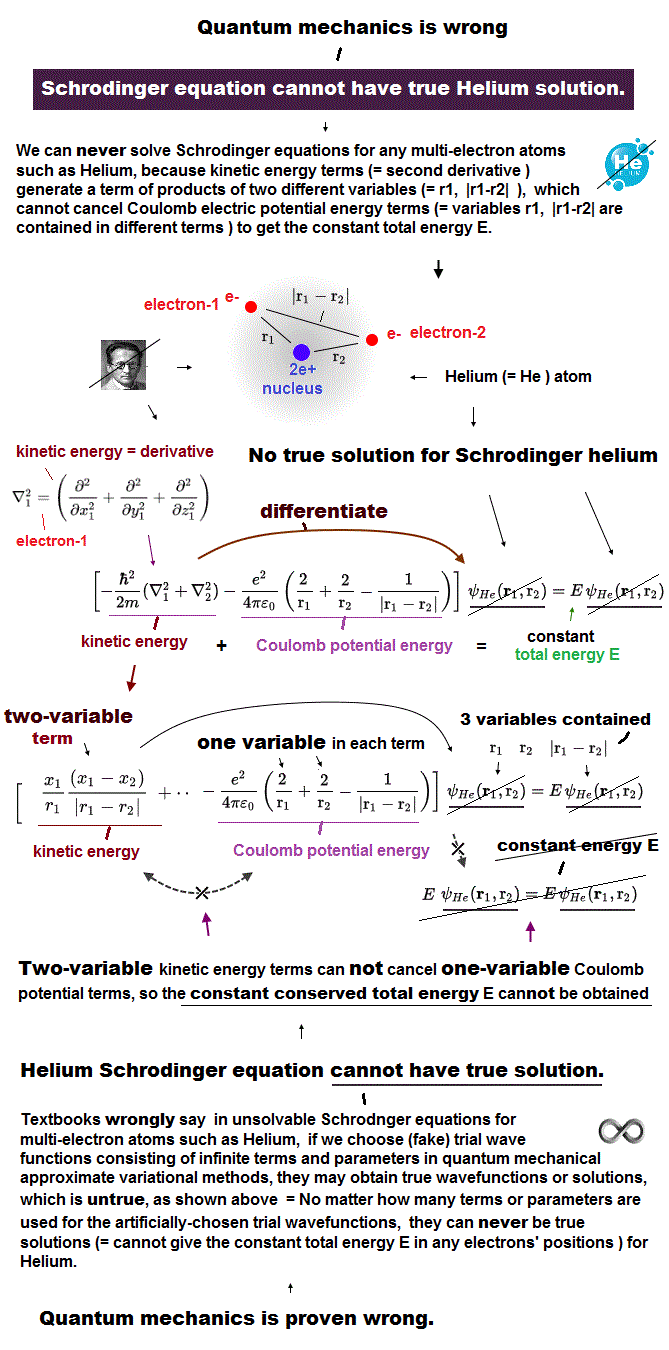Quantum mechanics relies on Schrödinger equation as the only calculation tool for getting (fake) atomic energies.

In any Schrödinger equations, the sum of electrons' kinetic energies and Coulomb potential energies must be equal to the total energy E, which energy value must be conserved and constant.

This total energy conservation law is the most important and inviolable physical principle also in quantum mechanical Schrödinger equations ( this p.2,  this p.2-1st-paragraph,  this p.1-last ).

For example, in order to obtain the true atomic wavefunction and energy for one-electron hydrogen atom, we have to solve the hydorgen atomic Schrödinger equation under the condition that the total energy E is constant and conserved = total energy E is Not affected or changed by electron's positions or coordinates (= x,y,z or r ).

To get the constant total energy E value, the Coulomb potential energy terms must be just canceled out by electron's kinetic energy term which kinetic energy is expressed as the second-order differential of atomic wavefunctions (= ∇2 ψ = (kinetic energy) × ψ ).

But in case of multi-electron atoms such as helium, Schrödinger equations can Not be solved under the condition of conserving the constant total energy E.

In two-electron helium atom, the electrons' kinetic energy terms expressed as the derivatives of helium's wavefunction (= ψ ) cannot cancel out the helium's three Coulomb potential energy terms ( this p.3,  this p.2 ).  ← Obtaining the conserved and constant total energy E value is impossible in helium's Schrödinger equation.

So in the unsolvable helium atom, we have to artificially choose fake solutions with many freely-adjustable parameters as a helium's approximate trial wavefunction and integrate the helium Schrödinger equation with the chosen wavefunction to obtain (fake) average helium energy.

This approximate pseudo-methods for unsolvable multi-electron Schrödinger equations can never give true atomic wavefunctions, due to violation of the total energy E (= the unsolvable helium's Schrödinger equation contains infinite numbers of different total energy E values inside a single atom, whose total energy E magically changes depending on electrons' positions or coordinates, which disagrees with the fact that only single definite helium's ground state total energy E value is observed. ).

Coulomb electric potential energies are expressed as functions (= -e2/4πε r ) inversely proportional to the electrons' variables (= r ) or coordinates (= x, y, z ).

On the other hand, the quantum mechanical kinetic energy is expressed as the second-order derivatives of wavefunction with respect to the electron's coordinate (x,y,z) or r , the kinetic energy operator = ∂^2/∂ x^2 + ∂^2/∂ y^2 + ∂^2/∂ z^2 or ∂^2/∂ r^2.. ( this p.2,  this p.2,  this p.3 ).

So the kinetic energy terms expressed as the second derivative could reduce the power or degree of the original wavefunction by two with respect to the electron's coordinate r  in comparison to the power or degree of Coulomb potential energy term (= inversely proportional to r ) is one-lower than the original wavefunction with respect to electron's coordinate r.

For such a kinetic energy term to cancel out Coulomb potential energy term and obtain the conserved and constant total energy E, each atomic wavefunction must be expressed as the product of the exponential function (= e-r ) and the polynomial function of r (= a + br + cr2 .. ).

↑ Because in order to cancel Coulomb potential energy term, one differential operator of the second-order differential kinetic energy operator must act on the exponential function of r (= No change of the power index of r in the exponential function ), and the remaining one differential operator of the second-order differential kinetic energy operator must act on the polynomial functions (= the power index r decreases by one ).

As a result, this kinetic energy term = second derivative of wavefunction consisting of the polynomial function multiplied by exponential function of the electron's coordinate r could reduce the power ( exponent ) of r of the original wavefunction by one and cancel Coulomb potential energy term (= -e2/4πε r ) whose power of r is one-lower (= Coulomb potential is inversely proportional to r ) than the original wavefunction.

When the kinetic energy's second differential operator acts only on the exponential function part twice, it causes no power or degree change, so it just gives the constant total energy value E ( × exponential function ) by the second differentiation.

When the kinetic energy's second differential operator acts only on the polynomial function part twice, it reduces the power of r by two, which must be canceled out by other kinetic energy terms such as angular kinetic energies (= ~l/r2), or the polynomial function becomes zero by second differential.

Only in one-electron hydrogen atoms, this mechanism of electron's kinetic energy expressed as the second derivative of wavefunctions canceling Coulomb potential energy term (= due to the same power 1/r between kinetic and Coulomb energies ) is working to give the constant and conserved total energy E value.  ← Only one-electron hydrogen atom is solvable.

But in a two-electron helium atom, this cancellation does Not work, hence, Schrödinger equations for helium can neither conserve the constant total energy E nor be solved.  ← Quantum mechanics is false.

In order to cancel three Coulomb potential energy terms between electron-1-nucleus, electron-2-nucleus and electron-1-electron-2, the hypothetical exact helium wavefunction must be the product of three sub-wavefunctions of three independent variables of r1 (= distance between electron-1 and nculeus ), r2 (= distance between electron-2 and nucleus ) and |r1-r2| (= or r12 = distance between electron-1 and electron-2 ).

So the kinetic energy terms expressed as derivatives of helium wavefunction ( ex. with respect to electron-1 coordinate r1 ) must always include the complicated kinetic energy terms of the product of 1/r1 × 1/|r1-r2|, which can Not be canceled by three Coulomb potential energy terms or any other kinetic energy terms.

↑ This problematic complicated kinetic energy term (= a/(r1×:|r1-r2| = product of two different independent variables r1 and |r1-r2| ), which can Not be canceled by any Coulomb energy term (= so the constant total energy E cannot be obtained ), is generated when one of the kinetic energy's second differential operator acts on the polynomial function f(r1), and the other differential operator acts on the polynomical function g(|r1-r2|).

As a result, Schrödinger equations for any multi-electron atoms such as helium and molecules can neither be solved nor conserve the constant total energy E, because the complicated kinetic energy terms consisting more than one independent variables (= r1 × |r1-r2| ) cannot be canceled by Coulomb potential energy terms (= each Coulomb term consists of only one variable, in the way -1/r1 - 1/r2 + 1/|r1-r2| ).

Of course, the differential of the variable |r1-r2| or r12 (= square root of (x1-x2)2+(y1-y2)2+(z1-z2)2 = distance between two electrons,  this p.5 ) with respect to r1 or (x1,y1,z1) always exposes far more complicated new variables (= not only the original variable r12, but also the x-component variable x1-x2 appear ), which can Not be eliminated.  ← Cancellation between kinetic and Coulomb potential energy terms' variables is impossible in a helium.  → Conserving the constant total energy E in a quantum mechanical helium is impossible.

This proves quantum mechanics is undeniably false.

The atomic ground state energies are described by time-independent Schrödinger equations, so the total energy E must be always conserved and constant (= it's impossoble that ghost energies suddenly pop out of nothing ), but paradoxical quantum mechanics obviously violates energy conservation law, so false.

## Schrödinger Helium doesn't exist.

### [ Two electrons must move "dependent" on another electron. ]

(Fig.7)  Each electron independent → Coulomb term independent → unreal atom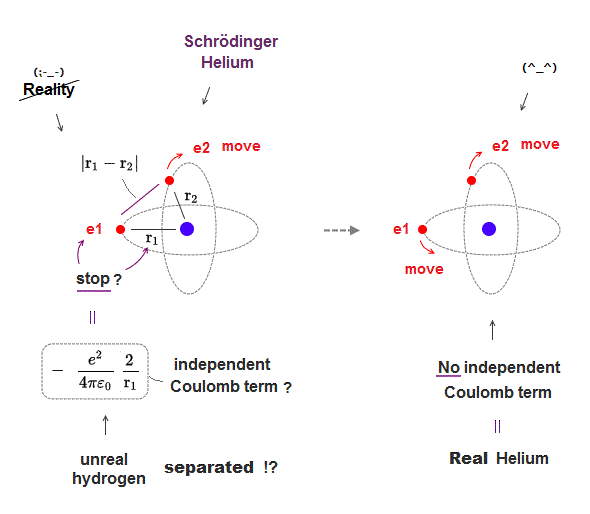For Helium to be real, two electrons must move with time, dependently on another electron, which prevents each Coulomb energy term from changing independently.

If two electrons can move independently, each Coulomb energy term must be independent, which splits Helium into unreal three hydrogens, which doesn't exist.

This is the reason Schrödinger equation is wrong in multi-electron atoms such as Helium, and only classical orbits can give real Helium model.

But in old 1920s, there were No computers, so they couldn't handle three-body Helium electrons' motion classically, which is the reason only wrong Schrödinger equation survived.

## Real atomic model.   → many molecules !

### [ Only Coulomb relation and de Broglie wave are enough. ]

(Fig.8)   Simple model describing real atomic force is necessary.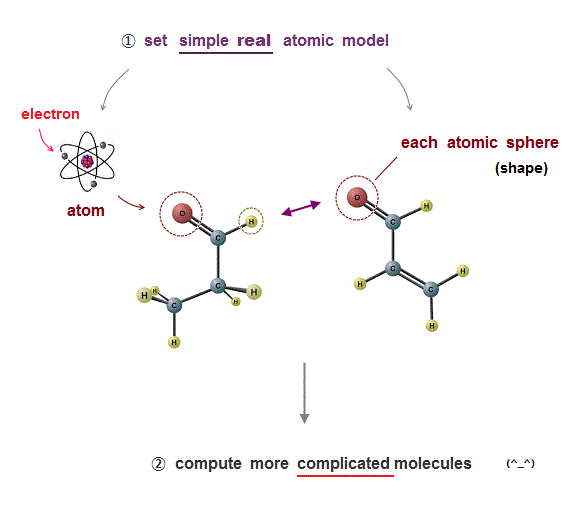We need only two conditions to describe all realistic molecular behavior.

If there is only Coulomb relation condition, atomic (elecron orbital) radius is not determined.

de Broglie wavelength determines atomic size, and it can explain the different sizes between carbon and silicon.

These two conditions can explain the maximum electrons' number (= Pauli principle ) correctly, without unreal spin.

This simple atomic model (= only Coulomb + de Broglie ) can be easily applied to much bigger, complicated molecules with the help of computer to cure fatal disease.

## Quantum mechanics is useless, forever.

### [ Schrödinger equation has No real electrons, so useless. ]

(Fig.9)   Simple useful model is impossible without real electrons.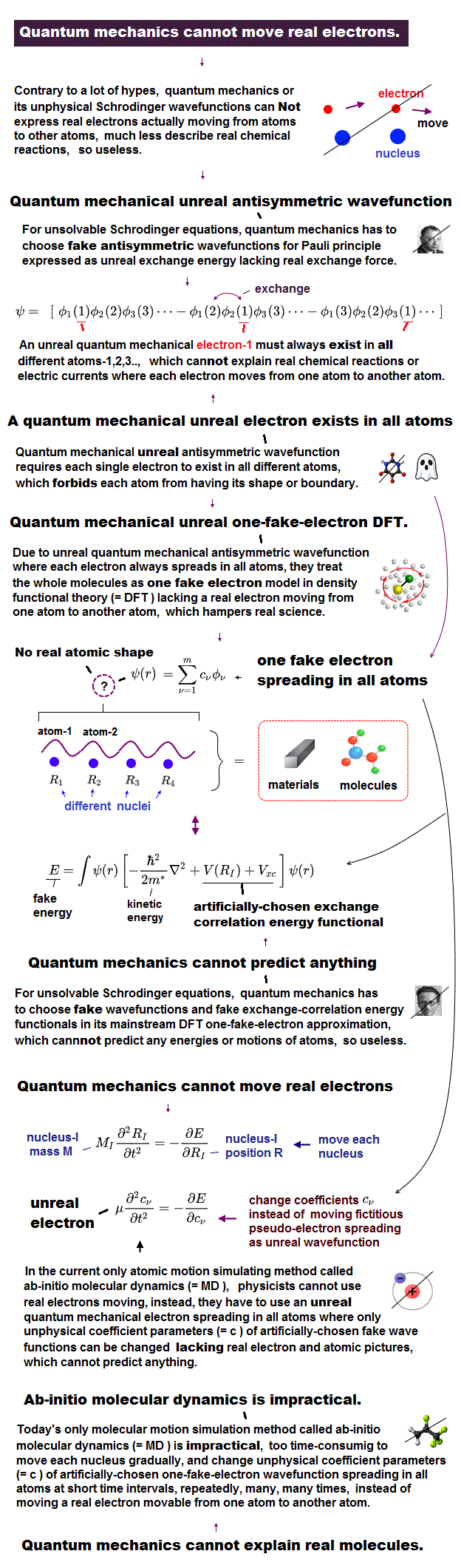As I proved above, it is impossible to find exact solution in multi-electron atoms in Schrödinger equation.

Quantum mechanics just chooses artificial fake solution in each atom, which method is completely useless.

In applied physics, they use density functional theory (= DFT ), which also chooses artificial fake Coulomb energy term V.

Each time we deal with different atoms, we have to choose different artificial fake solution in vain.

This is the reason why quantum mechanics is useless forever in applied science such as medicine, and it lacks reality.

## Only hydrogen atom is solvable.

### [ Quantum mechanics can solve only one-electron hydrogen. ]

(Fig.10)  Kinetic + potential energies = total energy E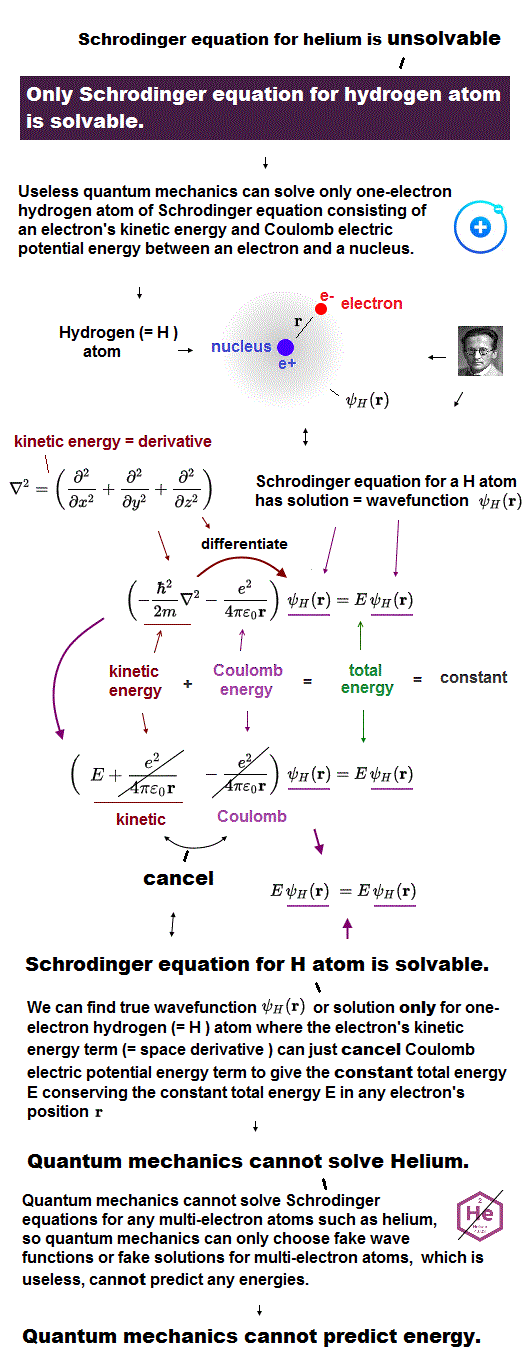Under the present science, Schrödinger equation in quantum mechanics is the only tool to calculate atomic energy.

Unfortunately, Schrödinger equation can solve only one-electron hydrogen atom, cannot deal with other multi-electron atoms.

Total energy E is the sum of an electron's kinetic energy and Coulomb potential energy in Schrödinger equation.

Of course, this total energy E must be conserved (= constant ) in every electron's position.

## Helium has No exact solution.

### [ Schrödinger equation of Helium cannot be solved. ]

(Fig.11)  ↓ Helium has No solution ( = No wave-function ψHe )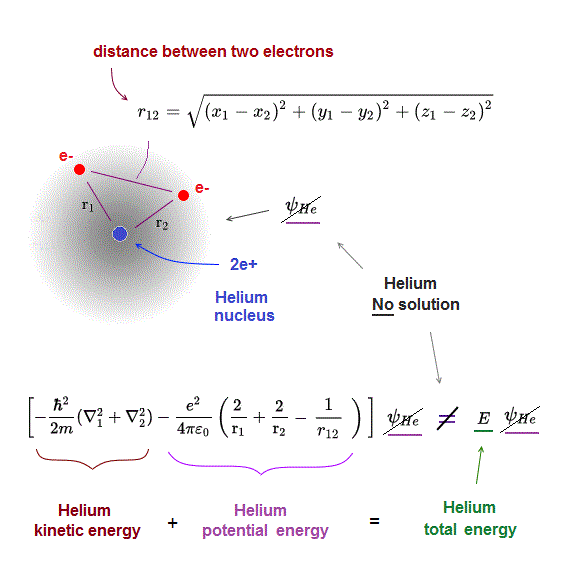Schrödinger equation of Helium with two electrons cannot be solved (= Schrödinger Helium has No exact solution ).

Helium potential energy consists of three terms.
Two of them are Coulomb attractive energies between each electron and Helium nucleus (= -2/r1,  -2/r2 in Fig.11 ).

The third term is Coulomb repulsive energy between two electrons.
It includes the variable r12, which represents the distance between two electrons.

## Choose approximate (fake) Helium solution.

### [ Helium solution consists of two hydrogen atoms ? ]

(Fig.12)  ↓ Helium approximate solution (= wavefunction )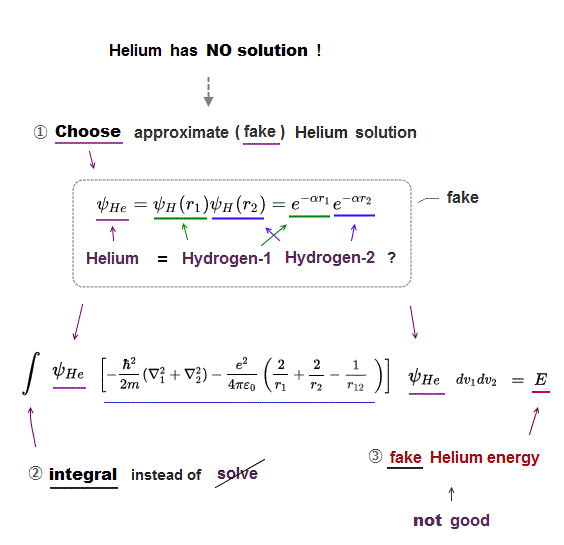Schrödinger equation of Helium cannot be solved.
So we need to choose some approximate (= fake ) Helium solution (= trial wavefunction ).

Ths simplest Helium solution consists of two hydrogen atoms 1 and 2.

After choosing the fake Helium solution and inserting it into Schrödinger equation, we integrate it instead of solving it ( this p.2 ).

By adjusting parameter α, the total (fake) energy E can be lowered.

But this simplest Helium solution cannot get exact Helium energy ( this calculated energy E becomes -77.4 eV which is higher than actual Helium energy -79.0 eV, see this p.4 ).

## Wrong trick in quantum mechanics.

### [ Quantum mechanics relies on "wrong math" in Helium. ]

(Fig.13)  ↓ Hylleraas (fake) Helium wavefunction includes r12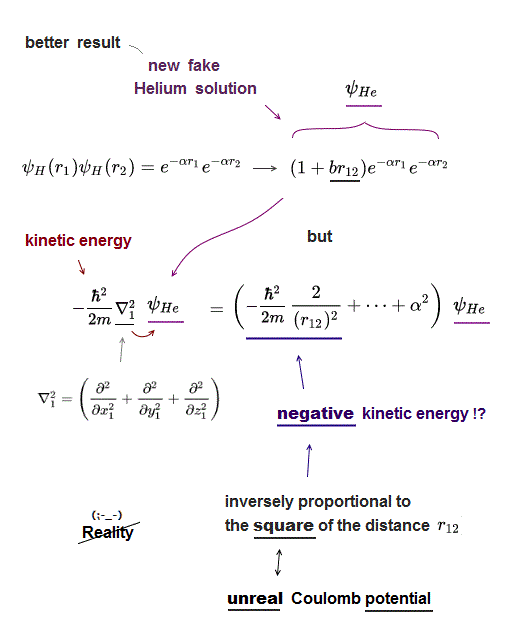To lower the Helium total energy, quantum mechanics has to rely on wrong math trick, which proves quantum mechanics is wrong.

Instead of the simplest approximate Helium solution (= just two hydrogens ), they use Hylleraas Helium solution which is a little more complicated including r12 (= distance between two electrons ).

This new fake Helium solution including r12 gives better (= lower ) total energy than the simplest solution ( this new Hylleraas solution gives -78.7 eV of Helium total energy, this p.8, which is still slightly higher than the actual Helium energy ).

But in fact, quantum mechanics depends on wrong math trick when this new Hylleraas Helium solution is used.

Kinetic energy of Schrödinger equation is expressed as the second derivative.

This kinetic energy term always gives "unreal negative kinetic energy" which is inversely proportional to the square of distance between two electrons ( ~ 1/r122).  ← this unusual term doesn't exist in the nature.

For the total energy to be conserved, the kinetic energy must cancel out the Coulomb potential energy which is inversely proportional to r12 (= 1/r12 ), Not to the square of r12 ( ~ 1/r122).

This is a wrong and unfair math trick, because the negative kinetic energy inversely propotronal to the square of r12 can unnaturally lower the Helium (fake) total energy in a wrong way.

Because it violates total energy conservation ( negative 1/r122 kinetic energy term cannot cancel Coulomb potential term 1/r12 ).

This is the trick by which quantum mechanics can seemingly get Helium energy value close to the experimental value.

But this deceptive quantum method sacrifices Helium total energy conservation (= kinetic energy + Coulomb potential energy cannot be constant E in this method )

Getting better energy value by deliberately violating total energy conservation (= lowering kinetic energy by dishonest method ) is physically false and inconsistent.

## The origin of negative kinetic energy.

### [ Dirty trick in quantum mechanics. ]

(Fig.14)  ↓ Hydrogen 2p wavefunction.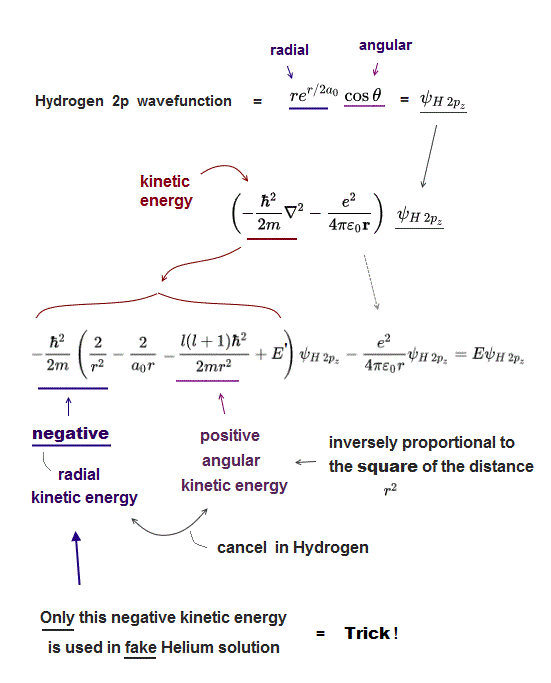Unsolvable Schrödinger equation of Helium relies on "dirty trick" to lower its total energy using "negative kinetic energy" inversely proportional to the square of distance r12.

Because negative kinetic energy term inversely proportional to the square of distance (= -1/r2) can be lower than the term inversely proportional to the distance (= -1/r  = Coulomb potential term ).

What's the origin of this nonphysical negative kinetic energy ?

This strange negative kinetic energy is necessary to cancel positive angular kinetic energy which increases inversely proportional to the square of distance (= 1/r2 ) in hydrogen 2p wavefunction.

Hydrogen 2p wavefunction consists of radial (= re-r ) and angular parts (= cosθ ).  See this p.5 angular momentum l=1.

Kinetic energy (= second derivative ∇2 ) of the angular part (= cosθ ) in Hydrogen 2p wavefunction becomes inversely proportional to the square of distance (= l(l+1)/r2,  see this p.5, this p.9 ).

For hydrogen total energy to be conserved (= E is constant ), this angular kinetic energy must be canceled out by corresponding negative kinetic energy inversely proportional to the square of r ( 0 = 1/r2-1/r2 ) in radial part.

Quantum mechanics uses only this radial part of negative kinetic energy in Hydrogen 2p wavefunction, omitting angular part (= spherical harmonics ) to lower Helium total energy in a dishonest way.

Of course, this Schrödinger Helium cannot conserve total energy E (= Not constant ), so physically wrong.  This is the dirty trick of quantum mechanics, which is Not "successful" at all.2018/8/20 updated. Feel free to link to this site.